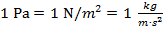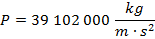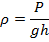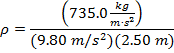# Pressure Formula

Pressure Formula

Pressure is a force per unit area that acts on an object. It can be expressed simply as P = F/A, where F is a force, and A is the area it acts on. Pressure is often calculated for gases and fluids. The pressure under a liquid or gas is equal to the density of that fluid multiplied by the acceleration due to gravity and the height (or depth) of the fluid above the certain point. The unit for pressure is the Pascal (Pa), andpressure = density of a fluid x acceleration due to gravity x height of fluid column

P = ρgh

P = pressure (Pa)

ρ = density of a gas or fluid (kg/m3)

g = acceleration due to gravity (9.80 m/s2)

h = the height of a column of gas or fluid (m)

Pressure Formula Questions:

1) The wreckage of the ship the Titanicis under 3800 m of ocean water. If the density of the cold saltwater above the Titanicis 1050 kg/m3, what is the pressure at that depth?

Answer: The pressure can be found using the formula:

P = ρgh

P = (1050 kg/m3)(9.80 m/s2)(3800 m)P = 39 102 000 Pa

P = 39.1MPa

The pressure of the ocean water at the depth of the Titanicis 39 102 000 Pa, which is approximately 39.1 MPa (mega-Pascal).

2) The pressure at the bottom of a cylinder that contains a gas is P = 735.0 Pa. If the height of the cylinder is 2.50 m, what is the density of the gas?

Answer: The density can be found by rearranging the pressure formula:ρ = 30.0 kg/m3

The density of the gas in the cylinder is 30.0 kg/m3.

 Related Links: Gases: Pressure Quiz Density Examples Pneumatics Examples